Numbers: Getting to grips with division

This free course is available to start right now. Review the full course description and key learning outcomes and create an account and enrol if you want a free statement of participation.

Free course

12 Dividing decimal numbers by moving the decimal point

Doing division when decimal numbers are involved is the same as doing divisions involving whole numbers, with a few extra steps to take care of the decimal point.

Either the number you are dividing into or the number you are dividing by, or both of them, may be a whole number or a decimal number. So, for example, you might want to do the following divisions:

• Example 1: 49.26457 ÷ 8
• Example 2: 2.601 ÷ 1.22
• Example 3: 678 ÷ 27.356

Before starting to do the division, you need to adjust the numbers so that the number you are dividing by is a whole number. In Example 1 above, the number you are dividing by (8) is already a whole number so you don’t need to do anything, even though the number you are dividing into (49.26457) is a decimal number.

In Examples 2 and 3 above, the number you are dividing by is a decimal number, so you do need to make adjustments. You have to:

1. Move the decimal point to the right in the number you are dividing by, until the divider becomes a whole number.
2. Count the number of places you have to move the decimal point in order to do this.
3. Move the decimal point in the number you are dividing into the same number of places to the right as you did in the number you are dividing by.

Look at Example 2 above. To make 1.22 into the whole number 122 you have to move the decimal point two places to the right. So you also move the decimal point two places to the right in 2.601, making it 260.1.

Active content not displayed. This content requires JavaScript to be enabled.
Interactive feature not available in single page view (see it in standard view).

Activity 6

Click on ‘Reveal discussion’ to see all the individual steps at once.

Discussion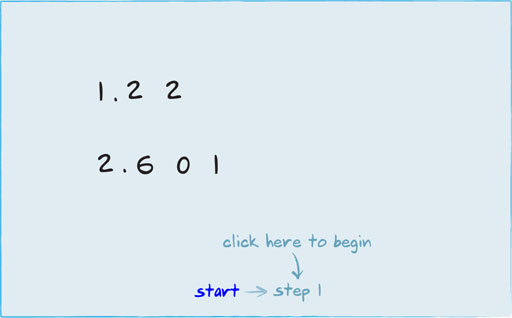Figure 29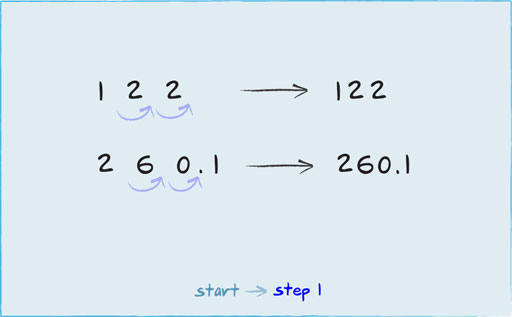Figure 30

In Example 3 above, you have to move the decimal point three places to the right to make the divider into the whole number 27356. But the number you are dividing into is already a whole number, so there is no decimal point to move. Click on each step below to see how the decimal point moves in Example 3.

Active content not displayed. This content requires JavaScript to be enabled.
Interactive feature not available in single page view (see it in standard view).

Activity 7

Click on ‘Reveal discussion’ to see all the individual steps at once.

Discussion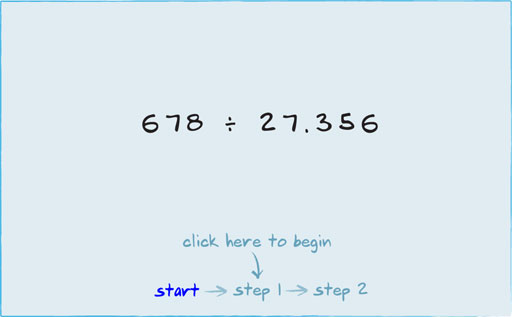Figure 31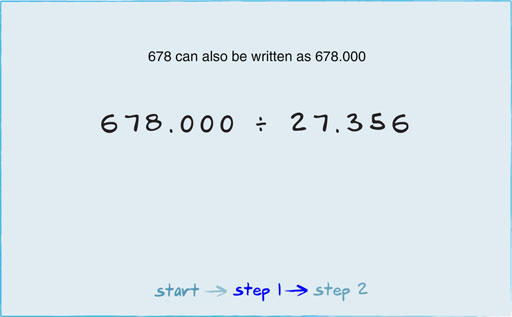Figure 32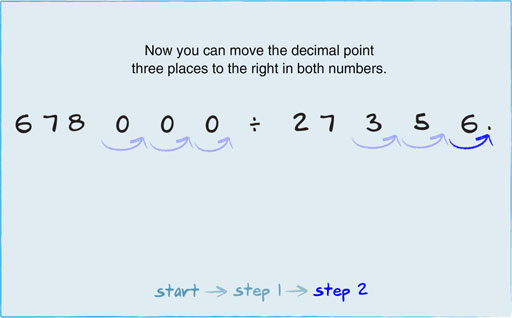Figure 33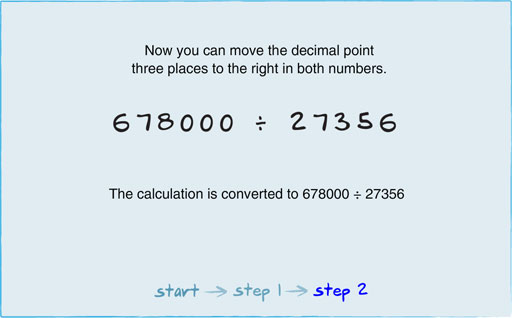Figure 34

Once the number you are dividing by is a whole number, you proceed with the division on paper in exactly the same way as when dealing entirely with whole numbers.

The only difference is that you ignore the decimal point in the number you are dividing into, if it still has one, until the end of the calculation. Then, to complete your answer, you insert the decimal point in the appropriate position. How you do this is shown in the next section.

Div_1